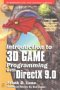# Maths - Bilinear Multiplication

We start by reviewing linearity and then extend this to bilineararity.

## Linear Multiplication.

To represent linear space we only need two operations:

• Vector addition: to add two vectors we take the start of the second vector and move it to the end of the first vector. The addition of these two vectors is the vector from the start of the first vector to the end of the second vector.
• Scalar multiplication changes the length of a vector without changing its direction. That is we 'scale' it by the multiplying factor. So scalar multiplication involves multiplying a scalar (single number) by a vector to give another number.

If the space is linear we have:

a*(v +u) = a*v + a*u

where:

• v & u are vectors
• a is a scalar

In other words the two operations are distributive.## Bilinear Multiplication

To have bilinearity we need to include a multiplication where both operands are vectors.For a bilinear multiplication, if we keep one of the vectors constant and then the other vector varies in a linear way, as defined above.

For example, in the diagram above we take the product as the area formed from the two vectors. So if the area formed from V and U is V•U then if we multiply V by a scalar value 'a' we get the area a*V•U, so,

(a*V)•U = a*(V•U)

and similarily if we multiply U by b:

V•(b*U) = b*(V•U)

so combining these gives:

(a*V)•(b*U) = ab*(V•U)

An example of this type of multiplication is the vector dot product.

However this type of multiplication takes two vectors as input and produces a scalar value as an output. Is it possible to have a bilinear multiplication which takes two vectors as input and produces a vector as an output?

The requirement is:

(a V + a' V')×(b U + b' U') = ab(V×U) + ab'(V×U') +a'b(V'×U) +a'b'(V'×U')

this applies to the vector cross product.

The vector cross product only applies in 1,3 and 7 dimensions. However there are ways to generalise the vector cross product to other numbers of dimensions.

 metadata block see also: Correspondence about this page Book Shop - Further reading. Where I can, I have put links to Amazon for books that are relevant to the subject, click on the appropriate country flag to get more details of the book or to buy it from them.Introduction to 3D Game Programming with DirectX 9.0 - This is quite a small book but it has good concise information with subjects like, maths introduction and picking.If you are interested in 3D games, this looks like a good book to have on the shelf. If, like me, you want to have know the theory and how it is derived then there is a lot for you here. Including - Graphics pipeline, scenegraph, picking, collision detection, bezier curves, surfaces, key frame animation, level of detail, terrain, quadtrees & octtrees, special effects, numerical methods. Includes CDROM with code. This book contains more mathematically rigorous methods for picking than described above. Other Math Books Specific to this page here: